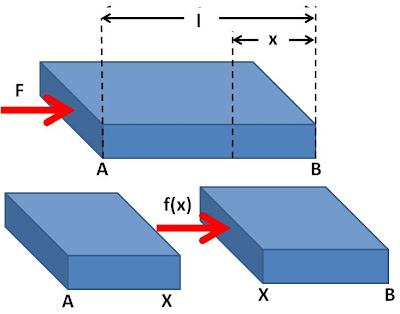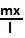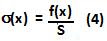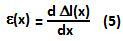## Wednesday, April 8, 2009

### Irodov Problem 1.295Suppose that the mass of the plank AB is m, its length is l and that is experiences an acceleration of a. Hence, we have,Now consider the motion of a section of the plank XB of length x. Since the plank is of uniform density, the mass of the section will be. Let force exerted by the plank section AX at the cross section of XB be f(x). This force f(x) is responsible for accelerating the plank section XB at rate a. Hence we have,The stress induced in the plank cross-section at point X is given by,Suppose that prior to the application of the force F, the plank was A'B, and the point X was located at X'. After the force, the plank gets compressed so that A moves to A' and X moves to X'. Let the change in length of section XB, XX' be. The strain experienced by the section XB of the plank is given by ,